# Comparison of Different Fin and Tube Compact Heat Exchanger with Longitudinal Vortex Generator in CFU-CFD Configurations

Comparison of Different Fin and Tube Compact Heat Exchanger with Longitudinal Vortex Generator in CFU-CFD Configurations
Department of Mechanical Engineering, Universidad de Chile, Beauchef 851, Santiago 8370456, Chile

Corresponding Author Email:
alvalenc@ing.uchile.cl
Page:
1523-1531
|
DOI:
https://doi.org/10.18280/ijht.390514
14 September 2021
|
Accepted:
15 October 2021
|
Published:
31 October 2021
| Citation

OPEN ACCESS

Abstract:

Over the last decades several studies have searched for improved Fin and Tube Heat Exchanger (FTHE) designs capable of providing the best thermo-hydraulic performance. The present study aims at quantifying and comparing the thermo-hydraulic performance of different FTHE configurations. Six different designs were analyzed. The first FTHE consisted of an in-line circular tube arrangement and the last one was a FTHE with staggered oval tube with two pairs of Delta Winglet Vortex Generators (DWVG) in common flow up–common flow down (CFU-CFD) configuration. The best performance was obtained using DWVG in CFU-CFD orientation. This configuration enabled a 90% increase of the thermal performance factor when compared with the first case, using only two pairs of vortex generator´s per tube.

Keywords:

Fin and Tube Heat Exchanger, longitudinal vortex generator, delta winglet, thermo-hydraulic performance

1. Introduction

Fin and tube compact heat exchanger (FTHE) is one of the most common types of compact heat exchanger. Commonly, FTHE uses fins on the air side to enhance compactness. The tubes can be individually finned or share one single continuous larger fin. FTHE can withstand high pressures on the tube side, and the operating temperature is limited by the type of bonding, materials employed and material thickness. Due to these characteristics, they are used in air-conditioning, refrigeration, airplanes, automobiles, heat pumps, among many other applications [1, 2].

Vortex Generators (VGs) can be employed as a way of increasing the heat transfer rate in FTHE. VGs are protrusions from a surface that intensify the heat transfer interrupting boundary layers, developing vortices, mixing flow and causing flow destabilization on the air side. There are two types of VGs: Transverse (TVG) and longitudinal vortex generators (LVG). TVG develop transverse vortices which are perpendicular to the flow direction, while LVG generate longitudinal vortices that last over long distances in the flow channel, resulting in a more efficient heat transfer enhancement [3, 4]. The angle of attack, aspect ratio and LVG design have a significant effect on heat transfer enhancement, . The delta winglet type is one of the most studied LVG, because causes a lower pressure loss when compared to other vortex generators .

Kwak et al. [6, 7] studied the effect of delta winglet VGs in staggered and in-line circular tubes arrangement. In their research, they built-in vortex generators with a common flow up (CFU) arrangement. Compared to the common flow down (CFD) configuration from Fiebig et al. , their experimental results showed larger heat transfer enhancement and lower pressure loss.

Changing the shape of the tubes is another passive technique. Fiebig et al.  conducted an experimental study where the local heat transfer with flat and circular staggered tubes was measured for Reynolds between 600 and 3000. They also investigated the influence of LVG and found that FTHE with flat tubes presented a better heat transfer performance than circular tubes.

Another tube shape consists of an oval type for FTHE. Han et al.  examined oval and different circular tubes through numerical research. In addition, they applied another passive technique consisting of two types of fins: louvered and wavy fin. They concluded that the oval geometry improved the flow characteristics in the wake region. This configuration reduces the flow resistance, achieving a uniform temperature distribution in the fin.

Chu et al.  carried out a study in FTHE using oval tubes and various number of tubes from 2 to 5 with LVG´s in form of delta winglets. The angle of attack of the LVG´s by 30° had the best performance. Additionally, Chu et al.  state a relation between the Nusselt number and the number of tubes. For a higher amount of tubes, the Nusselt number and the friction factor decreased. Therefore, the fewer the tubes per row, the better the performance.

Similarly, a number of studies have described a connection between the shape and geometric parameters of LVG and heat transfer enhancement. Gentry and Jacobi  suggested a direct dependency between vortex strength and Reynolds, wing aspect ratio and wing attack angle. Moreover, Díaz and Valencia  analyzed the impact of LVG shapes. In their research, they performed a numerical analysis to study heat transfer and pressure loss in a FTHE with oval tube and laminar flow. They compared the influence of different types of LVG: delta-type, rectangular, elliptical and spoon-type, the delta winglet type showed the highest increase in heat transfer.

A number of studies has suggested that the influence of LVG geometric parameters on the heat transfer and flow characteristics is correlated to the FTHE configuration. In an in-line circular tube and DWVG type configuration an angle of 45° showed the highest heat transfer enhancement for low Reynolds . Lei et al.  obtained different results, they compared different angles using Reynolds from 600 to 2600, but adding staggered circular tubes. Lei et al.  analyzed also the influence of the aspect ratio of the LVG, they combined these two parameters and concluded that for a two circular tube row with DWVG, the best performance was achieved with an aspect ratio of 2 and an angle of attack of 20°.

The amount of LVG in the flow channel also impacts the flow characteristics and heat transfer. Tiwari et al.  studied the impact of different configurations of DWVGs on heat transfer. In their research, they proposed various configurations of winglet pairs. The span-average Nusselt number was calculated and they concluded that the more LVG pairs, the better the heat transfer, along with a higher pressure loss. Recently, Carpio and Valencia  investigated a FTHE with a flat tube and multiple DWVGs, the configuration with 39 alternating LVG gave the highest thermal performance compared with the geometry without DWVGs with a 52% of increase on thermal-performance.

Chen et al.  combined CFD and CFU delta winglet VG orientation in an upstream configuration. They studied the effect of these arrangements in a fin and oval tube heat exchanger with an aspect ratio of 2, angle of attack of 30° and a fixed Reynolds of 300, LVG in staggered arrangement bring larger heat transfer enhancement than in in-line arrangement since the longitudinal vortices in the staggered arrangement influence a larger area and intensify the fluid motion normal to the flow direction. In the study of Salleh et al.  they have numerically investigated the thermal performance of LVG in CFD and CFU configuration in a model FTHE with four cylinders in line, LVG in CFU arrangement shows higher thermal and hydraulic performance for the three different LVG, however the base case without LVG was found to provide higher performance. This study shows that the increase in thermal performances using LVG is not always greater than one, and the optimal configuration for a FTHE depend on both cylinder and LVG arrangements and form. In the studies [4, 20] large numbers of compact heat exchanger studies have been compiled using VG until the year 2018. However, in the last year´s new developed in this field are reported.

Shi et al.  have reported the optimal location of curved DWVG in a FTHE model with circular cylinder in a staggered arrangement for obtain high thermal performance, the curved DWVG showed higher performance as the straight DWVG, the maximum performance was 26% by a Reynolds number of 1000, and decrease dramatically with an increase on the Reynolds numbers to turbulent region. Modi and Rathod  compare sinusoidal wavy and elliptical curved RWVG in a model of FTHE with in line circular tube arrangement, in term of the values of area goodness factor (j/f), the base case without LVG have the best performance. Concave and convex curved LGV have been compared by Song et al. , the thermal performance was not always greater than one and the effect of the cylinder was not considered. The effect of attack angle of concave and convex DWVG in a FTHE was investigated in Ref.  using the synergy principle, the thermal-performance decreases by 18% using the DWVG. Finally, H. Kobayashi et al.  have used topological optimization to obtain an optimal FTHE design using different LGV design, they obtain an area goodness factor (j/f) of 16% higher with the proposal design.

The purpose of this research is to investigate the enhancement between six different designs, to find out a superior performance, using a minimum quantitate of VGs pairs.

2. Model Description

2.1 Governing equations

The air is assumed to be incompressible with constant properties. Governing equations in the computational domain can be expressed as follows:

Continuity equation

$\frac{\partial u}{\partial x}+\frac{\partial v}{\partial y}+\frac{\partial w}{\partial z}=0$    (1)

Momentum equations

$\rho\left(\frac{\partial u}{\partial t}+u \frac{\partial u}{\partial x}+v \frac{\partial u}{\partial y}+w \frac{\partial u}{\partial z}\right)=-\frac{\partial P}{\partial x}+\mu\left(\frac{\partial^{2} u}{\partial x^{2}}+\frac{\partial^{2} u}{\partial y^{2}}+\frac{\partial^{2} u}{\partial z^{2}}\right)$    (2)

$\rho\left(\frac{\partial v}{\partial t}+u \frac{\partial v}{\partial x}+v \frac{\partial v}{\partial y}+w \frac{\partial v}{\partial z}\right)=-\frac{\partial P}{\partial y}+\mu\left(\frac{\partial^{2} v}{\partial x^{2}}+\frac{\partial^{2} v}{\partial y^{2}}+\frac{\partial^{2} v}{\partial z^{2}}\right)$    (3)

$\rho\left(\frac{\partial w}{\partial t}+u \frac{\partial w}{\partial x}+v \frac{\partial w}{\partial y}+w \frac{\partial w}{\partial z}\right)=-\frac{\partial P}{\partial z}+\mu\left(\frac{\partial^{2} w}{\partial x^{2}}+\frac{\partial^{2} w}{\partial y^{2}}+\frac{\partial^{2} w}{\partial z^{2}}\right)$    (4)

Energy equation

$\rho C_{p}\left(\frac{\partial T}{\partial t}+u \frac{\partial T}{\partial x}+v \frac{\partial T}{\partial y}+w \frac{\partial T}{\partial z}\right)=k\left(\frac{\partial^{2} T}{\partial x^{2}}+\frac{\partial^{2} T}{\partial y^{2}}+\frac{\partial^{2} T}{\partial z^{2}}\right)$    (5)

where,

$u\left[\frac{m}{s}\right], v\left[\frac{m}{s}\right], w\left[\frac{m}{s}\right], \rho\left[\frac{k g}{m^{3}}\right], t[s], P[P a], \mu\left[\frac{N s}{m^{2}}\right], C_{p}\left[\frac{J}{k g K}\right]$, $k\left[\frac{W}{m K}\right]$ represent the velocity in three directions, density, time, pressure, dynamic viscosity, specific heat and thermal conductivity, respectively.

2.2 Validation model

To validate the present work, Lei et al.  study was replicated. Their geometry was chosen because it represents a typical FTHE and they use DWVG with staggered circular tubes, as shown in Figure 1. The dimensions are shown in Table 1.

## 1.png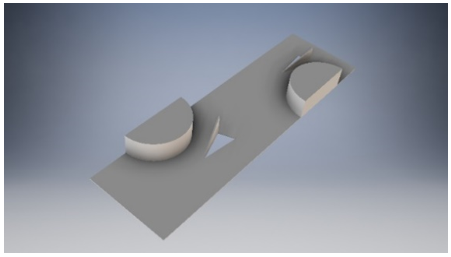Figure 1. FTHE design proposed by Lei et al. 

Table 1. FTHE geometric details 

 Dimension Value Transverse pitch (Tp) [mm] 12.7 Longitudinal pitch (Lp) [mm] 22 Fin pitch (Fp) [mm] 3.2 Fin thickness (Ft) [mm] 0.13 Tube outside diameter (D) [mm] 10.23 Air flow direction length (L) [mm] 44 DWVG angle of attack [°] 20 Aspect ratio 2

2.3 FTHE designs

In this study, six FTHE different designs were proposed based on a heat exchanger with three rows of tubes. Every case is more complex than its precursor, starting with a plane fin without DWVG. The geometric parameters of the proposed FTHE are similar to the parameters of the validation FTHE. The new dimensions are circular tube outside diameter (D) of 10.207 [mm], air flow direction length (L) 70 [mm], and DWVG angle of attack of 30 [°] in Figure 2.

## 2.png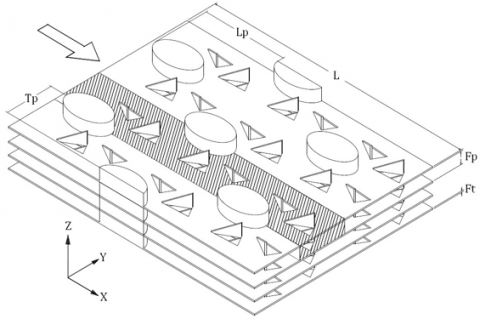Figure 2. FTHE design

## 3.png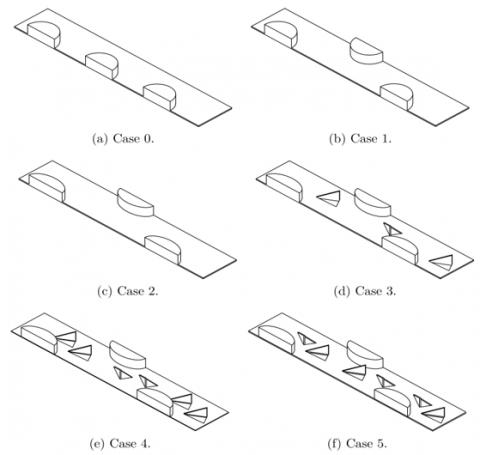Figure 3. FTHE designs

Figure 3 shows the six different investigated cases. Figure 3 (a) shows Case 0 with in-line circular tubes and no LVG, (b) shows the Case 1 with staggered circular tubes and no LVG, and (c) shows a FTHE with staggered oval tubes and no LVG. The difference between Case 1 and Case 2 is the geometry of the tubes, circular and oval tubes. In Figures 3 (d), (e) and (f) show a FTHE using staggered oval tubes with DWVG added in different quantities and orientations, in (d) only one pair of DWVG per tube in CFD orientation are used, in (e) two pairs of DWVG per tube in CFD-CFD orientation are added, and in (f) two pairs of DWVG per tube in CFU-CFD orientation are used.

The dimensions of the oval tubes were calculated based on the perimeter of the circular tubes, so the same heat transfer area was considered. In case 5, DWVG have the same parameters as DWVG in Case 4, changing only the orientation. Specific geometric dimensions are shown in Figures 4 and 5:

## 4.png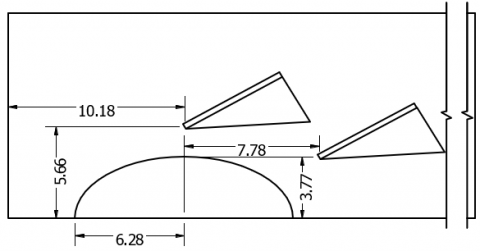Figure 4. Case 4 geometric details in millimeters

## 5.pngFigure 5. Case 5 geometric details in millimeters

2.4 Boundary conditions

The geometry was divided into three regions: upstream-extended, FTHE and downstream extended region. At the inlet boundary, uin the velocity and Tin the air temperature are constant. For upper, lower and lateral boundaries in extended regions, a symmetry condition was set. Outflow and periodic condition were applied at outlet and LVG region respectively.

## 6.pngFigure 6. Boundary conditions on the computational domain

At the LVG basis a periodic boundary condition was applied, to simulate the interaction between the fins with LVG. To ensure there was not any flow recirculation, the exit domain was also extended five times the length of the heat transfer region. The computational domain and boundary conditions can be seen in Figure 6 where the extended regions were shortened. The air inlet temperature is Tin=293 K, the fin and tube have a wall temperature of Twall=313 K, these temperatures are typically for FTHE. The inlet velocity was varied as 1.12 [m/s], 2.24 [m/s], 3.37 [m/s], 4.49 [m/s] and 5.61 [m/s] to obtain Reynolds number as 500, 1000, 1500, 2000 and 2500.

2.5 Parameters

The following relevant parameter definitions will be used in this investigation. The hydraulic diameter is defined in Eq. (6), considering Ao the total surface area, Ac is the minimum cross sectional area, and L is the air flow direction length .

$D_{H}=\frac{4 \cdot L A_{C}}{A_{o}}$    (6)

The Reynolds number is defined by the hydraulic diameter DH and is shown in Eq. (7), and the velocity Uc is the velocity at the minimum cross section area.

$R e=\frac{\rho \cdot U_{C} \cdot D_{H}}{\mu}$    (7)

where, $\rho$, $U_{C}$ and $\mu$ represent the density $\left[\frac{k g}{m^{3}}\right]$, velocity $\left[\frac{m}{s}\right]$ and dynamic viscosity $\left[\frac{\mathrm{kg}}{\mathrm{m} \cdot \mathrm{s}}\right]$, respectively.

The bulk temperature, as a function of the axial distance x, is shown in Eq. (8):

$T_{b u l k}(x)=\frac{\iint T(x, y, z) \cdot u(x, y, z) d y d z}{\iint u(x, y, z) d y d z}$    (8)

The log-mean temperature difference LMTD was calculated using the Eq. (9):

$L M T D=\frac{\left(T_{\text {wall }}-T_{\text {in }}\right)-\left(T_{\text {wall }}-T_{\text {out }}\right)}{\ln \frac{\left(T_{\text {wall }}-T_{\text {in }}\right)}{\left(T_{\text {wall }}-T_{\text {out }}\right)}}$    (9)

The average heat transfer coefficient can be determinate using Eq. (10):

$h=\frac{\dot{Q}}{A_{o} L M T D}$    (10)

$\dot{Q}$ is the total heat transfer rate [W], to determine the local Nusselt number in the fin, the bulk temperature and the heat flux at each point on the fin are used, $\dot{q}$ is reported by ANSYS Fluent 18.2 , as shown in Eq. (11).

$N u_{\text {local }}(x, z)=\left(\frac{\left.\dot{q}(x, z)\right| \quad _{y=0}}{T_{\text {wall }}-T_{\text {bulk }}\quad(x)}+\frac{\left.\dot{q}(x, z)\right| \quad _{y=T_{p}}}{T_{\text {wall }}-T_{\text {bulk }} \quad (x)}\right) \frac{D_{H}}{2 k}$    (11)

To determine the friction factor (f), the pressure difference and velocity Uc at the minimum cross section area are used, Eq. (12) shows the definition.

$f=\frac{2 \Delta P}{\rho \cdot U_{C}^{2}} \cdot \frac{A_{C}}{A_{0}}$    (12)

$\Delta P$ represent the pressure drop [Pa], to measure the efficiency of the increase in heat transfer when introducing LVG, the thermal performance JFw is used, as defined in Eq. (13), Carpio and Valencia . The value of the base geometry is the Case 0, therefore, when the factor JFw is greater than 1, it indicates an increase in efficiency with respect to the Case 0.

$J F_{w}=\frac{N u / N u_{o}}{\left(f / f_{o}\right)^{1 / 3}}$    (13)

3. Methodology

3.1 Numerical method

Ansys Fluent 18.2  was used to numerically simulate the different cases, the equations were iteratively solved using the finite volume method with SIMPLEC algorithm. The flow was considered laminar and we used transient simulations for a correct vortex development. The time step was set in ∆t = 105[s], and the number of time steps was selected so the simulation reached a stationary state. The convergence criterion for every time step was residuals less than 106 and 103 for energy and momentum equations. Second order for the equation discretization were used.

3.2 Grid generation

The grid was generated using two types of elements: Tetrahedrons, for the actual heat transfer region consisting of a complex geometry, and hex dominant elements for extended parts, which do not require a finer grid. To improve the quality of the mesh system, "body sizing" was applied. Smaller elements were used on the heat transfer region, and the smallest elements were inside "spheres of influence", which were centered in the VGs for a better flow behavior analysis.

The resulting grid consisted of almost 430,000 elements. To ensure an optimal mesh quality the orthogonal quality and skewness criteria were considered. The topology of the mesh is displayed in Figure 7.

## 7.png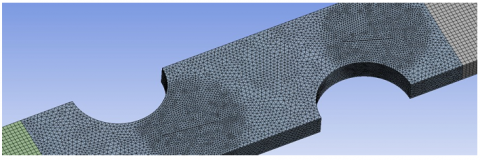Figure 7. Details of the mesh

3.3 Grid Independence

Three different meshes were analyzed, their characteristics are detailed in Table 2. The medium mesh is the one used in the validation model, and showed little variation compared with that by Lei et al. . The results obtained with the other meshes were compared with the medium grid and their values showed a difference of less than 5% on heat transfer coefficient and friction factor, thus proving the grid independence. The medium mesh provides precise results with lower computational times, and therefore was chosen.

Table 2. Details of the mesh

 Category Coarse Mesh Medium Mesh Fine Mesh Elements 210,755 428,232 863,125 Nodes 39,883 78,826 156,567 SOI’s elements size, mm 0.30 0.23 0.18 Max element size, mm 0.50 0.40 0.30

3.4 Validation results

## 8.png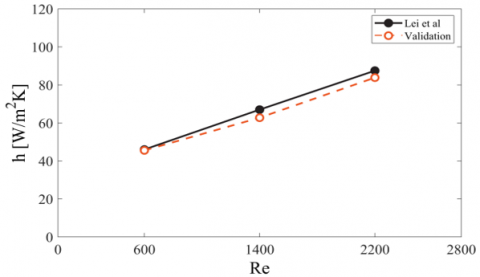Figure 8. Heat transfer coefficient model validation

## 9.png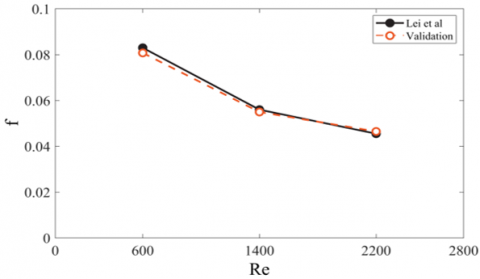Figure 9. Friction factor model validation

The present work was validated with the numerical results obtained by Lei et al. . To validate the results, the geometry described in Section 2.2 was simulated using the boundary conditions listed in Section 2.4. Fluid-solid conjugated heat transfer was modeled for the fin. The results of Lei et al.  was used as reference because they used a common geometry of FTHE without and with DWVG, and compare they numerical results with experimental data. Lei et al.  verify the numerical work with the model of FTHE without DWVG, they observed that the numerically predicted Colburn j-factor is about 1.5-7.8% higher than the experimental results, and the deviation of friction factor is between 1.6% and 9.2%.

The heat transfer coefficient and the friction factor values for Reynolds 600, 1400 and 2200 were compared. Both of them were calculated based on area averaged temperature using Eqns. (10) and (12) respectively. The Reynolds was expressed as Eq. (7).

The values obtained are shown in Figures 8 and 9. The results after the replication of Lei et al.  study showed a difference of less than 7% with the expected values, which is an acceptable difference.

4. Results

Figure 10 shows the velocity profile for the six investigated geometries without and with DWVG. A low velocity region can be recognized for Case 0, caused by the recirculation in the wake behind the tubes, this behavior inhibits the flow mix and does not contribute to the heat transfer. In the Case 1 the low velocity region after every tube is reduced because the tubes guide the flow into the wake region of the next row. In Case 2 staggered tubes are used but this time with oval shape, the change of shape has an impact on the wake region making it narrower so the low velocity region is even smaller than in Case 1.

In Case 3 DWVG generates longitudinal vortices that destabilize the flow and improve the mix even in dead water zones. In addition, because of their downstream position there is a smaller area for the air to flow between the DWVG and the tubes, which causes an increase in velocity as seen in Figure 10.

As a way of improving the heat transfer rate even more, two pairs of DWVG with CFD-CFD and CFU-CFD orientations were applied in Case 4 and Case 5, respectively. The higher the number of DWVG, the higher the flow mix. It is important to notice that, due to the generated longitudinal vortices the flow mix starts earlier on the channel, and their effect causes a thinner boundary layer after the third tube. In the Case 5 due the CFU-CFD DWVG orientation, the first DWVG (CFU) guides the flow to the next VG (CFD), resulting in a strong longitudinal vortex as seen in Figure 10.

The effects of DWVG on the temperature profile are showed in Figure 11 a), b) and c) for the Case 3, Case 4 and Case 5, respectively. DWVG produce a finer thermal boundary layer and mix the cold with the hot air and enhance the heat transfer after each DWVG. The Case 4 and 5 with two pairs of DWVG per cylinder showed the amplification of these effects on the thermal boundary layer.

## 10.png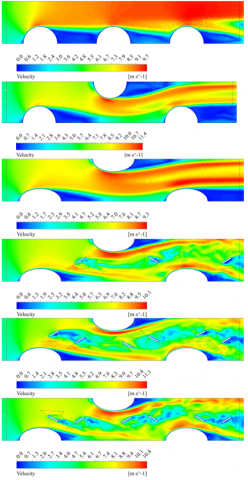Figure 10. Velocity profile for Case 0, Case 1, Case 2, Case 3, Case 4 and Case 5, Reynolds number Re=2000

## 11.png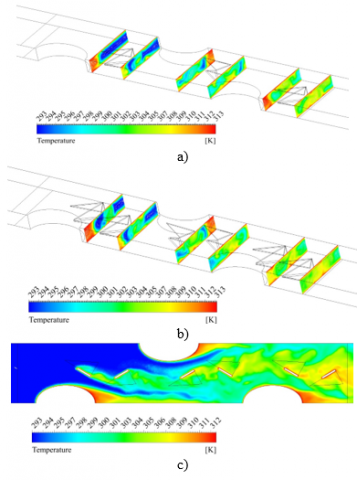Figure 11. Temperature profile for a) Case 3, b) Case 4 and c) Case 5, Reynolds number Re=2000

The span average Nusselt number is plotted in Figure 12. The staggered arrangement (Case 1, 2, 3, 4 and 5) clearly shows a better performance because the second tube in staggered arrangement guides the flow to the wake of the preceding tube. The first peak shows a quite similar behavior depending on the shape of the tube. The second peak begins as a consequence of the flow impact with the tube (for the cases without VGs). The curve in Case 2 reaches a lower second peak when compared to Case 1, but in Figure 13 the average Nusselt number is higher for Case 2. This implies that the heat transfer enhancement achieved thanks to the smaller stagnation zone of the first tube is greater than the increase in heat transfer caused by the horseshoe vortices generated by the second tube (which are stronger for the circular tube shape design). The presence of DWVG improves the span average Nusselt number due that the produced longitudinal vortices enhance the flow mix between tubes. Thus proving that the second and third peaks in cases 3, 4 and 5 are higher and also wider than in the previous cases 0, 1 and 2 without VGs.

## 12.png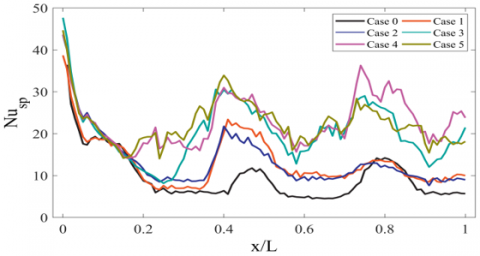Figure 12. Span-average Nusselt number for Reynolds number Re=2000

## 13.png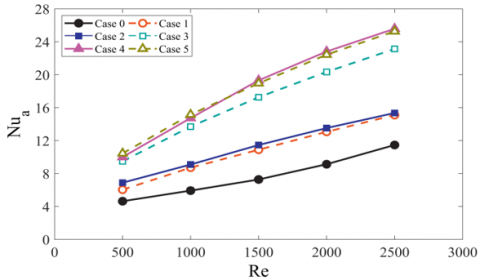Figure 13. Average Nusselt number

The average Nusselt number is plotted in Figure 13 and it was calculated based on the span average Nusselt number (Eq. 11), and the exact values are shown in Table 3. As expected, the Nusselt value increases with the Reynolds due to higher velocities, stronger vortices and better flow mix. For higher Reynolds the addition of a second pair of DWVG produces a better heat transfer enhancement than for lower Reynolds, improving the Nusselt number by up to 30% compared with the one-pair DVWG design. The average Nusselt number for different Reynolds is very similar for Cases 4 and 5, heat transfer enhancement is affected in a similar way by the strength of the vortices generated by CFU-CFD DWVG (Case 5) and the number of vortices produced by CFD-CFD DWVG (Case 4). The Reynolds number was varied until the onset of turbulence, for Reynolds number Re=2500 we have simulated using the k-ω turbulence model, and the average Nusselt number and friction factor were the same as using the laminar model.

The friction factor is presented in Figure 14, and the exact values are shown in Table 3. The in-line tube without DWVG exhibits the lowest friction factor among all the cases. There is only a slight difference between Case 1 and Case 2 in terms of f since both of them feature the same flow pattern. The augmentation of f in cases 3, 4 and 5 can be explained through the addition of DWVG; the longitudinal vortices generated produced an increase in the pressure loss. The higher the number of DWVG pairs, the greater the pressure loss. VGs orientation has an important effect on pressure loss, leading to an increment from 10% to 14% on the friction factor of the CFD-CFD configuration compared to CFU-CFD arrangement.

## 14.pngFigure 14. Friction factor

Table 3. Average Nusselt number and friction factor for Reynolds number

 Case Re Nu f Case 0 500 4.65 0.0331 1000 5.94 0.0234 1500 7.29 0.0194 2000 9.14 0.0169 2500 11.48 0.0157 Case 1 500 6.05 0.0458 1000 8.72 0.0344 1500 10.90 0.0287 2000 13.08 0.0252 2500 15.11 0.0229 Case 2 500 6.90 0.0485 1000 9.11 0.034 1500 11.47 0.0292 2000 13.53 0.0255 2500 15.37 0.0227 Case 3 500 9.49 0.0663 1000 13.71 0.05 1500 17.27 0.0426 2000 20.35 0.0382 2500 23.15 0.0345 Case 4 500 10.01 0.0793 1000 14.75 0.0622 1500 19.33 0.0557 2000 22.83 0.0505 2500 25.60 0.0458 Case 5 500 10.46 0.0718 1000 15.17 0.0564 1500 18.97 0.0489 2000 22.44 0.0445 2500 25.28 0.041

The JFw thermal-dynamic efficiency factor compared every geometry with the base case (Case 0) considering the effects of heat transfer and the associated pressure loss is showed in Figure 15. The best performance for every Reynolds studied is achieved in Case 5. The results obtained for the JFw factor shows that all the geometries have a higher efficiency as the FTHE with circular cylinder in-line. Every geometry showed a better performance than the preceding design for every Reynolds. The difference between the JFw factor in Case 4 and 5 is more substantial for lower Reynolds and the JFw factor converge into a value of 1.6 approximately for Reynolds 2500. Finally, the best performance is found in Case 5 for a Reynolds of 1500, where the JFw factor is 1.9 times larger than the first case.

## 15.png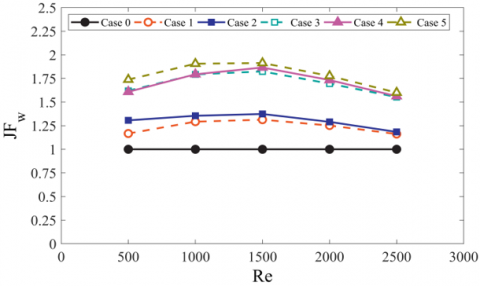Figure 15. JFw for different cases

5. Conclusions

The purpose of this study was to investigate the thermo-hydraulic performance of six different FTHE configurations. The best performance was obtained in a FTHE with staggered oval tubes and two pairs of DWVGs in CFU-CFD orientation. When compared with the first case (in-line circular tubes), showed an enhancement of 90% in the thermal performance.

The staggered arrangement improved the mix flow in recirculation zones compared with in line arrangement, because every tube guided the flow to the wake of the preceding tube. Oval tubes showed a better performance than circular tubes. Finally, the addition of DWVG showed the highest impact on the flow and heat transfer. Indeed, the implementation of one pair of VGs per tube increased the JFw by 30% in Case 3 compared to Case 2 which did not have any VGs.

Even though Case 4 -staggered oval tubes with two pairs of DWVG in CFD-CFD orientation- exhibited the higher average Nusselt number, the generated vortices also increased the pressure loss, reaching a higher pressure loss than with the CFU-CFD orientation, where fewer but stronger vortices were identified. The best performance is a balance between heat transfer and pressure loss and was found in the configuration with CFU-CFD DWVG, obtained a 90% of increase in thermal performance using two VGs pairs per tube.

Nomenclature
 Ac minimum cross sectional area, m2 A0 total surface area, m2 DH hydraulic diameter, m cp specific heat, J. kg-1. K-1 f friction factor g gravitational acceleration, m.s-2 h heat transfer coefficient, W/m2K k thermal conductivity, W.m-1. K-1 JFw thermal performance L air flow direction length, m. LMTD log-mean temperature difference, K Nusp span-average Nusselt p pressure, Pa $\dot{q}$ heat flux, W/m2 $\dot{Q}$ total heat transfer rate, W Re Reynolds number t time, s T temperature, K u velocity, m/s v velocity, m/s w velocity, m/s x coordinate, m y coordinate, m z coordinate, m Greek symbols ρ Density, kg/m3 $\mu$ dynamic viscosity, Ns/m2
References

 Sekulic, D.P. (2018). Compact heat exchangers. In: Kulacki F. (eds) Handbook of Thermal Science and Engineering. Springer, Cham, pp. 1-20. https://doi.org/10.1007/978-3-319-32003-8_36-1

 Thulukkanam, K. (2013). Heat Exchanger Design Handbook, Second Edition. CRC Press.

 Fiebig, M. (1997). Vortices and heat transfer. ZAMM Zeitschrift für Angewandte Mathematik und Mechanik. 77(1): 3-18. https://doi.org/10.1002/zamm.19970770103

 Chai, L., Tassou, S.A. (2018). A review of airside heat transfer augmentation with vortex generators on heat transfer surface. Energies, 11(10): 2737. https://doi.org/10.3390/en11102737

 Biswas, G., Torii, K., Fujii, D., Nishino, K. (1996). Numerical and experimental determination of flow structure and heat transfer effects of longitudinal vortices in a channel flow. International Journal of Heat and Mass Transfer, 39(16): 3441-3451. https://doi.org/10.1016/0017-9310(95)00398-3

 Kwak, K.M., Torii, K., Nishino, K. (2003). Heat transfer and pressure loss penalty for the number of tube rows of staggered finned-tube bundles with a single transverse row of winglets. International Journal of Heat and Mass Transfer, 46(1): 175-180. https://doi.org/10.1016/S0017-9310(02)00235-1

 Kwak, K.M., Torii, K., Nishino, K. (2005). Simultaneous heat transfer enhancement and pressure loss reduction for finned-tube bundles with the first or two transverse rows of built-in winglets. Experimental Thermal and Fluid Science, 29(5): 625-632. https://doi.org/10.1016/j.expthermflusci.2004.08.005

 Fiebig, M., Valencia, A., Mitra, N.K. (1993). Wing-type vortex generators for fin-and-tube heat exchangers. Experimental Thermal and Fluid Science, 7(4): 287-295, https://doi.org/10.1016/0894-1777(93)90052-K

 Fiebig, M., Valencia, A., Mitra, N.K. (1994). Local heat transfer and flow losses in fin and-tube heat exchangers with vortex generators: A comparison of round and flat tubes. Experimental Thermal and Fluid Science, 7(2): 130. https://doi.org/10.1016/0894-1777(93)90118-3

 Han, H., He, Y.L., Li, Y.S., Wang, Y., Wu, M. (2013). A numerical study on compact enhanced fin-and-tube heat exchangers with oval and circular tube configurations. International Journal of Heat and Mass Transfer, 65: 686-695. https://doi.org/10.1016/j.ijheatmasstransfer.2013.06.049

 Chu, P., He, Y.L., Lei, Y.G., Tian, L.T., Li, R. (2009). Three-dimensional numerical study on fin-and-oval-tube heat exchanger with longitudinal vortex generators. Applied Thermal Engineering, 29(5-6): 859-876. https://doi.org/10.1016/j.applthermaleng.2008.04.021

 Gentry, M.C., Jacobi, A.M. (2002). Heat transfer enhancement by delta-wing generated tip vortices in flat-plate and developing channel flows. Journal of Heat Transfer, 124(6): 1158-1168. https://doi.org/10.1115/1.1513578

 Díaz, D., Valencia, A. (2017). Heat transfer in an oval tube heat exchanger with different kinds of longitudinal vortex generators. Heat Transfer Research, 48(18): 1707-1725. https://doi.org/10.1615/HeatTransRes.2017018543

 Wu, J.M., Tao, W.Q. (2007). Investigation on laminar convection heat transfer in fin and-tube heat exchanger in aligned arrangement with longitudinal vortex generator from the viewpoint of field synergy principle. Applied Thermal Engineering, 27(14-15): 2609-2617. https://doi.org/10.1016/j.applthermaleng.2007.01.025

 Lei, Y.G., He, Y.L., Tian, L.T., Chu, P., Tao, W.Q. (2010). Hydrodynamics and heat transfer characteristics of a novel heat exchanger with delta-winglet vortex generators. Chemical Engineering Science, 65(5): 1551-1562. https://doi.org/10.1016/j.ces.2009.10.017

 Tiwari, S., Maurya, D., Biswas, G., Eswaran, V. (2003). Heat transfer enhancement in cross-flow heat exchangers using oval tubes and multiple delta winglets. International Journal of Heat and Mass Transfer, 46(15): 2841-2856. https://doi.org/10.1016/S0017-9310(03)00047-4

 Carpio, J., Valencia, A. (2020). Heat transfer enhancement through longitudinal vortex generators in compact heat exchangers with flat tubes. International Communications in Heat and Mass Transfer, 120: 105035. https://doi.org/10.1016/j.icheatmasstransfer.2020.105035

 Chen, Y., Fiebig, M., Mitra, N.K. (2000). Heat transfer enhancement of finned oval tubes with staggered punched longitudinal vortex generators. International Journal of Heat and Mass Transfer, 43(3): 417-435. https://doi.org/10.1016/S0017-9310(99)00157-X

 Salleh, M., Gholami, A., Wahid, M.A. (2019), Numerical evaluation of thermal hydraulic performance in fin-and–tube heat exchangers with various vortex generator geometries arranged in common-flow-down or common–flow-up. Journal of Heat Transfer, 141(2): 021801. https://doi.org/10.1115/1.4041832

 Awais, M., Bhuiyan, A.A. (2018). Heat transfer enhancement using different types of vortex generators (VGs): A review on experimental and numerical activities. Thermal Science and Engineering Progress, 5: 524-545. https://doi.org/10.1016/j.tsep.2018.02.007

 Shi, W.N., Liu, T.F., Song, K.W., Zhang, Q., Hu, W.L., Wang, L.B. (2021). The optimal longitudinal location of curved winglets for better thermal performance of a finned-tube heat exchanger. International Journal of Thermal Sciences, 167: 107035. https://doi.org/10.1016/j.ijthermalsci.2021.107035

 Modi, A., Rathod, M (2019). Comparative study of heat transfer enhancement and pressure drop for fin-and-circular compact heat exchangers with sinusoidal wavy and elliptical rectangular winglet vortex generator, International Journal of Heat and Mass Transfer, 141: 310-326. https://doi.org/10.1016/j.ijheatmasstransfer.2019.06.088

 Song, K.W., Tagawa, T., Chen, Z.H., Zhang, Q. (2019). Heat transfer characteristics of concave and convex vortex generators in the channel of plate exchanger under laminar flow. International Journal of Thermal Sciences, 137: 215-228. https://doi.org/10.1016/j.ijthermalsci.2018.11.002

 Syaiful, Yunianto, B., Salsabila, C.D., Fajar T.K., F., Soetanto, M.F. (2021). Effect of attack angle of concave and convex winglets vortex generators on thermal-hydraulic performance of fin and tube heat exchangers with field synergy principle, International Journal of Heat and Technology, 39(3): 797-809. https://doi.org/10.18280/ijht.390313

 Kobayashi, H., Yaji, K., Yamasaki, S., Fujita, K. (2019). Freeform winglet design of fin-and-tube heat exchangers guided by topology optimization. Applied Thermal Engineering, 161: 114020. https://doi.org/10.1016/j.applthermaleng.2019.114020

 ANSYS Fluent documentation, 2020. https://www.ansys.com/products/fluids/ansys-fluent.